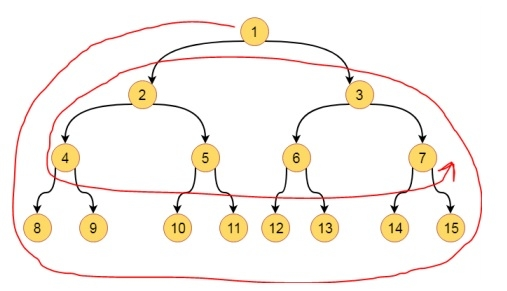# Anti Clockwise spiral traversal of a binary tree?

CServer Side ProgrammingProgramming

Here we will see one interesting problem. We have one binary tree. We have to traverse the tree in anti-clockwise manner. The traversal will be like below −The traversal sequence is 1, 8, 9, 10, 11, 12, 13, 14, 15, 3, 2, 4, 5, 6, 7

## Algorithm

#### antiClockTraverse(root)

Begin
i := 1, j := height of the tree
flag := false
while i <= j, do
if flag is false, then
print tree elements from right to left for level i
flag := true
i := i + 1
else
print tree elements from left to right for level j
flag := false
j := j - 1
end if
done
End

## Example

#include<iostream>
using namespace std;
class Node {
public:
Node* left;
Node* right;
int data;
Node(int data) { //constructor to create node
this->data = data;
this->left = NULL;
this->right = NULL;
}
};
int getHeight(Node* root) {
if (root == NULL)
return 0;
//get height of left and right subtree
int hl = getHeight(root->left);
int hr = getHeight(root->right);
return 1 + max(hl, hr); //add 1 for root
}
void printLeftToRight(class Node* root, int level) {
if (root == NULL)
return;
if (level == 1)
cout << root->data << " ";
else if (level > 1) {
printLeftToRight(root->left, level - 1);
printLeftToRight(root->right, level - 1);
}
}
void printRightToLeft(struct Node* root, int level) {
if (root == NULL)
return;
if (level == 1)
cout << root->data << " ";
else if (level > 1) {
printRightToLeft(root->right, level - 1);
printRightToLeft(root->left, level - 1);
}
}
void antiClockTraverse(class Node* root) {
int i = 1;
int j = getHeight(root);
int flag = 0; //flag is used to change direction
while (i <= j) {
if (flag == 0) {
printRightToLeft(root, i);
flag = 1; //set flag to print from left to right
i++;
}else {
printLeftToRight(root, j);
flag = 0; //set flag to print from right to left
j--;
}
}
}
int main() {
struct Node* root;
root = new Node(1);
root->left = new Node(2);
root->right = new Node(3);
root->left->left = new Node(4);
root->left->right = new Node(5);
root->right->left = new Node(6);
root->right->right = new Node(7);
root->left->left->left = new Node(8);
root->left->left->right = new Node(9);
root->left->right->left = new Node(10);
root->left->right->right = new Node(11);
root->right->left->left = new Node(12);
root->right->left->right = new Node(13);
root->right->right->left = new Node(14);
root->right->right->right = new Node(15);
antiClockTraverse(root);
}

## Output

1 8 9 10 11 12 13 14 15 3 2 4 5 6 7